# Module 1 - Great Minds

## Transcription

Module 1 - Great Minds
```Eureka Math™ Homework Helper
2015–2016
Grade 8
Module 1
Lessons 1–13
Eureka Math, A Story of Ratios®
Published by the non-profit Great Minds.
Copyright © 2015 Great Minds. No part of this work may be reproduced, distributed, modified, sold, or
commercialized, in whole or in part, without consent of the copyright holder. Please see our User Agreement for
more information. “Great Minds” and “Eureka Math” are registered trademarks of Great Minds.
20
8•1
-1
6
A Story of Ratios
15
Homework Helper
G8-M1-Lesson 1: Exponential Notation
Use what you know about exponential notation to complete the expressions below.
(−2) × ⋯ × (−2) = (−)
1. �����������
35 times
9

9
2. �
� × ⋯× � � = � �
���������
2
12 times
2

When the base (the number being repeatedly
multiplied) is negative or fractional, I need to use
parentheses. If I don’t, the number being multiplied
won’t be clear. Some may think that the 2 or only
the numerator of the fraction gets multiplied.
3. ��
8 ×���
⋯×
��
8 = 856
_____ times
The exponent states how many times the  is multiplied. It is multiplied  times so that is what is
written in the blank.
4. Rewrite each number in exponential notation using 3 as the base.
a.
b.
c.
d.
9 =  ×  =
27 =  ×  ×  =
81 =  ×  ×  ×  =
243 =  ×  ×  ×  ×  =
All I need to do is figure out how
many times to multiply 3 in order to
get the number I’m looking for in
parts (a)–(d).
5. Write an expression with (−2) as its base that will produce a negative product.
One possible solution is shown below.
(−)
= (−) × (−) × (−) = −
Lesson 1:
© 2015 Great Minds eureka-math.org
G8-M1-HWH-1.3.0-08.2015
Exponential Notation
To produce a negative product, I need
to make sure the negative number is
multiplied an odd number of times.
Since the product of two negative
numbers results in a positive product,
multiplying one more time will result
in a negative product.
1
20
8•1
-1
6
A Story of Ratios
15
Homework Helper
G8-M1-Lesson 2: Multiplication of Numbers in Exponential Form
Let , , and  be numbers and  ≠ 0. Write each expression using the fewest number of bases possible.
1. (−7)3 ∙ (−7)4 =
(−)+
2 7
3
I have to be sure that the
base of each term is the
same if I intend to use
the identity   ∙   =
+ for Problems 1–4.
2 5
3
3. � � ∙ � � =
+

� �
5.  3 ∙ 4  5 =

2.

∙  ∙  ∙  =
+ ∙ +
7.
2 5
2
=
+
4.
24 ∙ 8 2 =

∙ � � =
++
6.
(−3)5
(−3)2
27
32

=
=
−
−
Lesson 2:
=
(−)−

For this problem, I
know that 8 = 23 , so
I can transform the 8
to have a base of 2.
∙  ∙  =
8.
∙  =
© 2015 Great Minds eureka-math.org
G8-M1-HWH-1.3.0-08.2015
In this problem, I see
two bases,  and .
I can use the
commutative
property to reorder
the ’s so that they
are together and
reorder the ’s so
they are together.
5 ∙  6 =
Multiplication of Numbers in Exponential Notation
When the bases are the
same for division
problems, I can use the

identity  =  − .
The number 27 is
the same as
3 × 3 × 3 or 33 .
2
8•1
6
-1
A Story of Ratios
15
20
Homework Helper
G8-M1-Lesson 3: Numbers in Exponential Form Raised to a
Power
Lesson Notes
Students will be able to rewrite expressions involving powers to powers and products to powers. The
following two identities will be used:
For any number  and any positive integers  and , (  ) =   .
For any numbers  and  and positive integer , () =     .
Show (prove) in detail why (3 ∙  ∙ )5 = 35 ∙  5 ∙  5 .
( ∙  ∙ ) = ( ∙  ∙ ) ∙ ( ∙  ∙ ) ∙ ( ∙  ∙ ) ∙ ( ∙  ∙ ) ∙ ( ∙  ∙ )
= ( ∙  ∙  ∙  ∙ ) ∙ ( ∙  ∙  ∙  ∙ ) ∙ ( ∙  ∙  ∙  ∙ )
=  ∙  ∙
In the lesson today, we learned
to use the identity to simplify
these expressions. If the
directions say show in detail, or
prove, I know I need to use the
identities and properties I knew
before this lesson to show that
the identity I learned today
actually holds true.
By the definition of exponential
notation
By the commutative and
associative properties
By the definition of exponential notation or by the first law of exponents
=
If I am going to use the first law of
exponents to explain this part of my
proof, I might want to show another
line in my work that looks like this:
31+1+1+1+1 ∙  1+1+1+1+1 ∙ 1+1+1+1+1.
Lesson 3:
© 2015 Great Minds eureka-math.org
G8-M1-HWH-1.3.0-08.2015
Numbers in Exponential Form Raised to a Power
3
8•1
6
-1
A Story of Ratios
15
20
Homework Helper
G8-M1-Lesson 4: Numbers Raised to the Zeroth Power
Let , , , and  be numbers (, , ,  ≠ 0). Simplify each of the following expressions.
1.
6
6
2.
= −
=
=
3. 45 ∙
1
45

=

= −
=
=
5.
In class, I know we
defined a number raised
to the zero power as 1.
I have to use the rule
for multiplying
fractions.
I multiply the
numerator times the
numerator and the
denominator times the
denominator.
4 ∙3
3 ∙4
= − −
=
=  ∙
=
4. 37 ∙
1
35
∙ 35 ∙
1
37
∙ 32 ∙
0

= � � ∙ � �
= ∙ ∙ ∙
= − ∙ −
=
=  ∙
=
=  ∙
=  ∙
=
Lesson 4:
1
32
∙  ∙  ∙  ∙  ∙
∙  ∙
++

= ++

=

= −
=
=
=
6. �82 (26 )�
∙
=
∙
© 2015 Great Minds eureka-math.org
G8-M1-HWH-1.3.0-08.2015
3  4
3  4
Numbers Raised to the Zeroth Power
There is a power
outside of the
grouping symbol.
That means I
must use the
third and second
laws of exponents
to simplify this
expression.
4
8•1
6
-1
A Story of Ratios
15
20
Homework Helper
G8-M1-Lesson 5: Negative Exponents and the Laws of Exponents
Lesson Notes
You will need your Equation Reference Sheet. The numbers in parentheses in the solutions below correlate to
the reference sheet.
Examples
1. Compute: (−2)4 ⋅ (−2)3 ⋅ (−2)−2 ⋅ (−2)0 ⋅ (−2)−2
= (−)++(−)++(−)
= (−)
= −
Negative exponents follow the
same identities as positive
exponents and exponents of
zero.
2. Without using (10), show directly that ( −1 )6 =  −6 .

�− � = ��
By definition of negative exponents (9)

By � � =  (14)
= −
By definition of negative exponents (9)
=

3. Without using (13), show directly that
−

= − ⋅
=

=

+
=
=

=
⋅

= 6−12 .
By product formula for complex fractions

By definition of negative exponents (9)

⋅
By product formula for complex fractions
By  ∙  = + (10)

= −
By definition of negative exponents (9)
Lesson 5:
© 2015 Great Minds eureka-math.org
G8-M1-HWH-1.3.0-08.2015
6−9
63
Negative Exponents and the Laws of Exponents
5
8•1
6
-1
A Story of Ratios
15
20
Homework Helper
G8-M1-Lesson 6: Proofs of Laws of Exponents
Lesson Notes
You will need your Equation Reference Sheet. The numbers in parentheses in the solutions below correlate
to the reference sheet.
Examples
1. A very contagious strain of bacteria was contracted by two
people who recently travelled overseas. When the couple
returned, they then infected three people. The next week,
each of those three people infected three more people.
This infection rate continues each week. By the end of 5
weeks, how many people would be infected?
Week of Return
+
Week 1
( × ) + ( + )
� × � + ( × ) + ( + )
Week 2
� × � + � × � + ( × ) + ( + )
Week 3
The 3 people infected upon
return each infect 3 people.
Therefore, in week 1, there are 9
new infected people, or
(3 × 3) = 32 .
Those 9 people infect 3 people
each, or 27 new people.
(32 × 3) = 33
� × � + � × � + � × � + ( × ) + ( + )
Week 4
� × � + � × � + � × � + � × � + ( × ) + ( + )
Week 5
2. Show directly that  −10 ∙  −12 =  −22 .

− ⋅ − =  ∙

=  ⋅

By definition of negative exponents (9)
By product formula for complex fractions
= +
By  ∙  = + for whole numbers  and  (6)
= −
By definition of negative exponents (9)

=
Lesson 6:
© 2015 Great Minds eureka-math.org
G8-M1-HWH-1.3.0-08.2015
Proofs of Laws of Exponents
“Show directly”
and “prove”
mean the same
thing: I should
use the identities
and definitions I
know are true for
whole numbers
to prove the
identities are
true for integer
exponents also.
6
1. What is the smallest power of 10 that would exceed 6,234,579?
has  digits, so a number with  digits will exceed it.
6
G8-M1-Lesson 7: Magnitude
8•1
-1
A Story of Ratios
15
20
Homework Helper
If I create a number with the
same number of digits as
but with all nines, I know that
number will exceed . If I
then add 1, I will have a
number that can be written as
a power of 10.
= , ,  < , ,  < , ,  =
The smallest power of  that would exceed , ,
is  .
2. Which number is equivalent to 0.001: 103 or 10−3? How do you know?
− is equivalent to . . Positive powers of  create large numbers, and negative powers of
create numbers smaller than one. The number − is equal to the fraction

,

which is the same as
and . . Since .  is a small number, its power of  should be negative.
3. Jessica said that 0.0001 is bigger than 0.1 because the first number has more digits to the right of the
decimal point. Is Jessica correct? Explain your thinking using negative powers of 10 and the number line.
.  =

= − and .  =

= − . On a number
line − is closer to  than − ; therefore, − is larger
than − .
4. Order the following numbers from least to greatest:
102
10−4
100
10−3
− < − <  <
Lesson 7:
© 2015 Great Minds eureka-math.org
G8-M1-HWH-1.3.0-08.2015
I have to remember that
negative exponents behave
differently than positive
exponents. I have to think
about the number line and that
the further right a number is,
the larger the number is.
Since all of the bases are the same, I just
need to make sure I have the exponents in
order from least to greatest.
Magnitude
7
8•1
6
-1
A Story of Ratios
15
20
Homework Helper
G8-M1-Lesson 8: Estimating Quantities
1. A 250 gigabyte hard drive has a total of 250,000,000,000 bytes of available storage space. A 3.5 inch
double-sided floppy disk widely used in the 1980’s could hold about 8 × 105 bytes. How many doublesided floppy disks would it take to fill the 250 gigabyte hard drive?
, , ,  ≈  ×
×
= ×

×
I know that when the question
says, “How many will it take to
fill…,” it means to divide.
= .  × −
= .  ×
= ,
It would take ,  floppy disks to fill the  gigabyte hard drive.
2. A calculation of the operation 2,000,000 × 3,000,000,000 gives an answer of 6  + 15. What does the
answer of 6  + 15 on the screen of the calculator mean? Explain how you know.
The answer means  ×  . This is known because
� ×  � × �  ×  � = ( × ) × � ×  �
=  × +
=  ×
I know that multiplication follows
the commutative and associative
properties. I can then use the
first law of exponents to simplify
the expression.
3. An estimate of the number of neurons in the brain of an average rat is 2 × 108 . A cat has approximately
8 × 108 neurons. Which animal has a greater number of neurons? By how much?
×  >  ×
I need to divide to figure out how
many times larger the cat’s number
of neurons are than the rat’s.
×
= ×
×
=  × −
=  ×
=  ×
=
The cat has  times as many neurons as the rat.
Lesson 8:
© 2015 Great Minds eureka-math.org
G8-M1-HWH-1.3.0-08.2015
Estimating Quantities
8
8•1
6
-1
A Story of Ratios
15
20
Homework Helper
G8-M1-Lesson 9: Scientific Notation
Definitions
A positive decimal is said to be written in scientific notation if it is expressed as a product  × 10 , where  is a decimal
greater than or equal to 1 and less than 10 and  is an integer.
The integer  is called the order of magnitude of the decimal  × 10 .
Examples
1. Write the number 32,000,000,000 in scientific notation.
, , ,  = .  ×
= �.  ×  � + � .  × � ×  ��
= �.  ×  � + ��.  ×  � ×  �
= �.  ×  � + � ×  �
= (.  + ) ×
= (.  ×  ) ×

= .  ×
Lesson 9:
© 2015 Great Minds eureka-math.org
G8-M1-HWH-1.3.0-08.2015
By first law of exponents
By associative property of multiplication
By distributive property
= .  ×
Scientific Notation
I will need to multiply 3.2 by 1010
because I need to write an equivalent
form of 32,000,000,000.
To add terms, they need to be like
terms. I know that means that the
magnitudes, or the powers, need to
be equal.
2. What is the sum of 5.4 × 107 and 8.24 × 109 ?
�.  ×  � + �.  ×  �
I will place the decimal between the 3
and 2 to achieve a value that is greater
than 1 and smaller than 10.
I know that “× 102 ” multiplies 8.24
by 100.
The last step is to write this in
scientific notation.
9
3. The Lextor Company recently posted its quarterly earnings for 2014.
Quarter 1: 2.65 × 106 dollars
Quarter 2: 1.6 × 108 dollars
Quarter 3: 6.1 × 106 dollars
Quarter 4: 2.25 × 108 dollars
What is the average earnings for all four quarters? Write your answer in scientific notation.
�.  ×  � + �.  ×  � + �.  ×  � + �.  ×  �
=

�.  ×  � + �.  ×  ×  � + �.  ×  � + �.  ×  ×  �
=

�.  ×  � + � ×  � + �.  ×  � + � ×  �
=

(.  +  + .  + ) ×
=

.  ×
=

.
=
×

= .  ×
= .  ×
The average earnings in 2014 for the Lextor Company is .  ×  dollars.
Lesson 9:
© 2015 Great Minds eureka-math.org
G8-M1-HWH-1.3.0-08.2015
Scientific Notation
10
6
8•1
-1
A Story of Ratios
15
20
Homework Helper
G8-M1-Lesson 10: Operations with Numbers in Scientific
Notation
1. A lightning bolt produces 1.1 × 1010 watts of energy in about 1 second. How much energy would that
bolt of lightning produce if it lasted for 24 hours? (Note: 24 hours is 86,400 seconds.)
�.  ×  � × ,
= �.  ×  � × �.  ×  �

= (.  × . ) × �
= .  × +
I need to take the amount of energy
produced in one second and multiply
it by 86,400.

×  �
= .  ×
A lightning bolt would produce .  ×  watts of energy if it lasted  hours.
2. There are about 7,000,000,000 people in the world. In Australia, there is a population of about
2.306 × 107 people. What is the difference between the world’s and Australia’s populations?
, , ,  − .  ×
= � ×  � − �.  ×  �

= � ×  ×  � − �.  ×  �
= � ×  � − �.  ×  �
= ( − . ) ×
Just like in the last lesson, I need to
make sure the numbers have the
same order of magnitude
(exponent) before I actually
subtract.
= .  ×
= .  ×
The difference between the world’s and Australia’s populations is about .  ×  .
Lesson 10:
© 2015 Great Minds eureka-math.org
G8-M1-HWH-1.3.0-08.2015
Operations with Numbers in Scientific Notation
11
6
8•1
-1
A Story of Ratios
15
20
Homework Helper
3. The average human adult body has about 5 × 1013 cells. A newborn baby’s body contains approximately
2.5 × 1012 cells.
a.
Find their combined cellular total.
Combined Cells = � ×  � + �.  ×  �
= � ×  ×  � + �.  ×  �
= � ×  � + �.  ×  �
= ( + . ) ×
= .  ×
= .  ×
The combined cellular total is .  ×  cells.
b.
Given that the number of cells in the average elephant is approximately 1.5 × 1027, how many
human adult and baby combined cells would it take to equal the number of cells of an elephant?
.
.  ×
=
×
.  ×  .
≈ .  × −
= .  ×
= .  ×
It would take .  ×  adult and baby combined cells to equal the number of cells of an
elephant.
Lesson 10:
© 2015 Great Minds eureka-math.org
G8-M1-HWH-1.3.0-08.2015
Operations with Numbers in Scientific Notation
12
6
8•1
-1
A Story of Ratios
15
20
Homework Helper
G8-M1-Lesson 11: Efficacy of Scientific Notation
1. Which of the two numbers below is greater? Explain how you know.
8.25 × 1015 and 8.2 × 1020

The number .  ×  is greater. When comparing each
numbers order of magnitude, it is obvious that  > ;
therefore, .  ×  > .  ×  .
To figure out which number is
greater, I need to look at the order
of magnitude (exponent) of each
number.
2. About how many times greater is 8.2 × 1020 compared to 8.25 × 1015 ?
.
.  ×
=
×
.  ×  .
= . … × −
≈ .  ×
= ,
.  ×  is about ,  times greater than .  ×  .
3. Suppose the geographic area of Los Angeles County is 4,751 sq. mi. If the state of California has area
1.637 × 105 square miles, that means that it would take approximately 35 Los Angeles Counties to make
up the state of California. As of 2013, the population of Los Angeles County was 1 × 107 . If the
population were proportional to area, what would be the population of the state of California? Write
your answer in scientific notation.
×  ×  =  ×
= (.  × ) ×
= .  × � ×  �
= .  ×
The population of California
is .  ×  .
Lesson 11:
© 2015 Great Minds eureka-math.org
G8-M1-HWH-1.3.0-08.2015
Since it takes about 35 Los Angeles Counties to
make up the state of California, then what I
need to do is multiply the population of Los
Angeles County by 35.
The expression 35 × 107 is not in scientific
notation because 35 is too large (it has to
be less than 10). I can rewrite 35 as 3.5 ×
10 because 35 = 3.5 × 10.
Efficacy of Scientific Notation
13
6
8•1
-1
A Story of Ratios
15
20
Homework Helper
G8-M1-Lesson 12: Choice of Unit
1. What is the average of the following two numbers?
To find the average, I need to add
the two numbers and then divide
by 2. Since the numbers are raised
to the same power of 10, I really
only need to add 3.257 and 3.1.
3.257 × 103 and 3.1 × 103
The average is
.  ×  + .  ×  (.  + . ) ×
=

.  ×
=

.
=
×

= .  ×
2. Assume you are given the data below and asked to decide on a new unit in order to make comparisons
and discussions of the data easier.
1.9 × 1015
3.75 × 1019
4.56 × 1017
2.4 × 103
9.26 × 1016
a.
b.
7.02 × 1019
I need to examine the exponents to see
which is most common or which exponent
most numbers would be close to. Since
I’m deciding the unit, I just need to make
sure my choice is reasonable.
What new unit would you select? Name it and express it using a power of 10.
I would choose to use  as my unit. I’m ignoring the number with  because it is so much
smaller than the other numbers. Most of the other numbers are close to  . I will name my unit
.
Rewrite at least two pieces of data using the new unit.
.×

= .  × − = .  × − = .
.  ×
.×

rewritten in the new unit is . .
= .  × − = .  ×  = .
.  ×  rewritten in the new unit is . .
Lesson 12:
© 2015 Great Minds eureka-math.org
G8-M1-HWH-1.3.0-08.2015
Choice of Unit
To rewrite the data, I will
take the original number
and divide it by the value of
my unit, , which is 1018 .
14
6
8•1
-1
A Story of Ratios
15
20
Homework Helper
G8-M1-Lesson 13: Comparison of Numbers Written in Scientific
Notation and Interpreting Scientific Notation Using Technology
1. If  × 10 <  × 10 , what are some possible values for  and ? Explain how you know.
When two numbers are each raised to the same power of , in this case the power of , then you only
need to look at the numbers  and  when comparing the values (Inequality (A) guarantees this). Since
we know that  ×  <  ×  , then we also know that  < . Then a possible value for  is  and
a possible value for  is  because  < .
2. Assume that  × 10−5 is not written in scientific notation and  is positive. That means that  is greater
than zero but not necessarily less than 10. Is it possible to find a number  so that
× 10−5 < 1.1 × 105 is not true? If so, what number could  be?
Since − = .  and .  ×  = , then
a number for  bigger than .  ×  would show that
× − < .  ×  is not true.
If  = .  ×  , then by substitution
× − = �.  ×  � × −
= .  × +(−)
= .  ×  .
If  = .  ×  , then  × − = .  ×  . Therefore
can be any number as long as  > .  × .
If  × 10−5 < 1.1 × 105 is not
written in scientific notation, it
means that  can be a really
large number. What I’m really
being asked is if there is a
number I can think of that
when multiplied by 10−5 or its
equivalent
1
100000
would be
larger than 1.1 × 105?
3. Which of the following two numbers is greater?
2.68941 × 1027 or 2.68295 × 1027
Since .  > . , then
.  ×  > .  ×  .
Lesson 13:
© 2015 Great Minds eureka-math.org
G8-M1-HWH-1.3.0-08.2015
Since both numbers are raised to the
same power (Inequality (A) again), all
I need to compare is 2.68941 and
2.68295.
Comparison of Numbers Written in Scientific Notation and Interpreting
Scientific Notation Using Technology
15
6
8•1
-1
A Story of Ratios
15
20
Homework Helper
```

### Meaningful quotes - Bentley University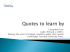More information

### Mathematics Curriculum 8 Integer Exponents and Scientific Notation Table of Contents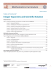More information

### File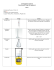More information

### February - Stapleton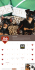More information

### Laboratory Math I: Exponents, Units and Scientific Notation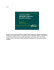More information

### 1.4 | Order of Operations (BEDMAS)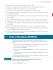More information

### Resume - KM Reps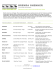More information

### Chaos in the Swinging Atwood Machine (SAM)More information

### Scientific notation and standard form classnotes.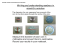More information

### The History and Evolution of the Musical Symbol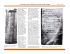More information

### Drum Set Notation in Logic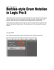More information

### pg 2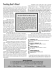More information

### Swaralipi: A Framework for Transcribing and Rendering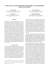More information